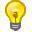• 当用户访问 web 页面时，他的名字可以记录在 cookie 中。document.cookie 将以字符串的方式返回所有的 cookies，类型格式： cookie1=value; cookie2=value; cookie3=value;

{
var d = new Date();
d.setTime(d.getTime()+(exdays*24*60*60*1000));
var expires = "expires="+d.toGMTString();
document.cookie = cname + "=" + cvalue + "; " + expires;
}

{
var name = cname + "=";
for(var i=0; i<ca.length; i++)
{
var c = ca[i].trim();
if (c.indexOf(name)==0) return c.substring(name.length,c.length);
}
return "";
}

{
{
}
else
{
{
}
}
}

## 实例

```<div class="content-bg">
<div class="content-intro view-box "> <h2>JavaScript <span class="color_h1">Cookies</span></h2> <script>
{
if (fname==null) {fname="";}
if (fname!="") {fname="firstname="+fname;}
if (lname==null) {lname="";}
if (lname!="") {lname="; lastname="+lname;}